# Volatility, Variance & Python¶

Exercises in Computational Finance

Yves Hilpisch

yves@pythonquants.com

www.pythonquants.com

The Python Quants GmbH

10th Fixed Income Conference, Barcelona, 26. September 2014

• Founder and MD of The Python Quants GmbH
• Lecturer Mathematical Finance at Saarland University
• Co-organizer of "For Python Quants" conference in New York
• Organizer of "Python for Quant Finance" meetup in London
• Organizer of "Python for Big Data Analytics" meetup in Berlin
• Book (2015) "Derivatives Analytics with Python", Wiley Finance
• Book (2014) "Python for Finance", O'Reilly
• Dr.rer.pol in Mathematical Finance
• Martial Arts Practitioner and Fan

See www.hilpisch.com.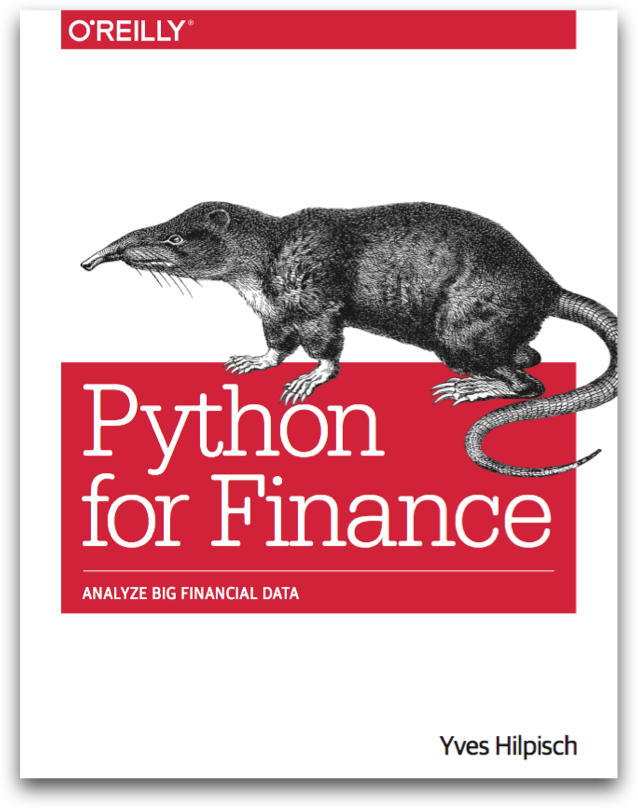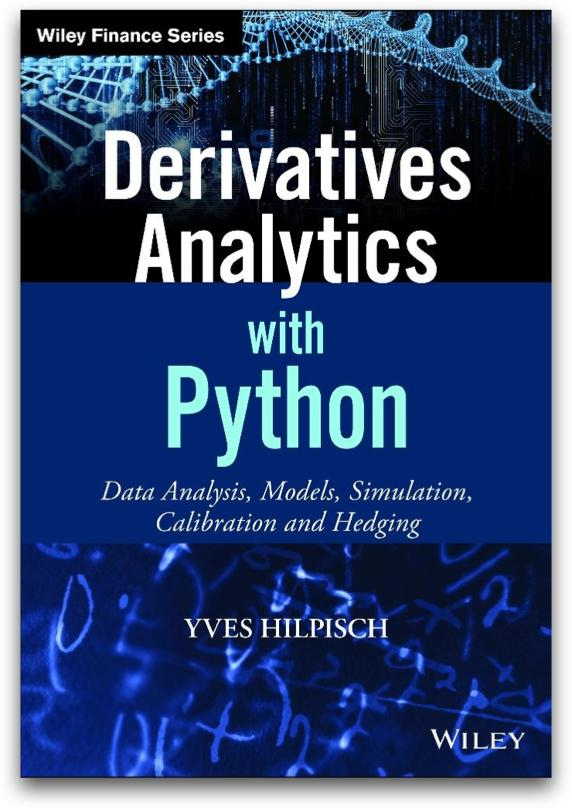## The Python Quants¶

• The Python Quants GmbH, Germany
• The Python Quants LLC., New York City
• Consulting, Development & Training
• Python and Quantitative Finance
• Python Quant Platform http://quant-platform.com
• DEXISION – GUI-based Financial Engineering www.dexision.com
• DX Analytics – Python-based derivatives analytics library

## Agenda¶

This talk/tutorial is about new approaches for the marketing and modelling of listed volatility and variance products. The focus lies on the VSTOXX volatility index and related derivatives as well as on the just launched Variance Futures by Eurex. Topics include:

• Derivatives Analytics
• DX Analytics
• Python Quant Platform
• VSTOXX Options Pricing

## Derivatives Analytics¶

### Historical Milestones¶

Important Milestones (I), from a rather personal perspective.

• Bachelier (1900) – Option Pricing with Arithmetic Brownian Motion
• Einstein (1905) – Rigorous Theory of Brownian Motion
• Samuelson (1950s) – Systematic Approaches to Option Pricing
• Black Scholes Merton (1973) – Geometric Brownian Motion, Analytical Option Pricing Formula
• Merton (1976) – Jump Diffusion, Option Pricing with Jumps
• Boyle (1977) – Monte Carlo for European options
• Cox Ross Rubinstein (1979) – Binomial Option Pricing

Important Milestones (II), from a rather personal perspective.

• Harrison Kreps Pliska (1979/1981) – General Risk-Neutral Valuation, Fundamental Theorem of Asset Pricing
• Cox Ingersoll Ross (1985) – Intertemporal General Equilibrium, Short Rates with Square-Root Diffusion
• Heston (1993) – Stochastic Volatility, Fourier-based Option Pricing
• Bates (1996) – Heston (1993) plus Merton (1976)
• Gruenbichler Longstaff (1996) – Volatility Futures and Options Pricing
• Bakshi Cao Chen (1997) – Bates (1996) plus Cox Ingersoll Ross (1985)
• Carr Madan (1999) – Using Fast Fourier Transforms for Option Pricing
• Longstaff Schwartz (2001) – Monte Carlo for American Options
• Gamba (2003) – Monte Carlo for (Complex) Portfolios of Interdependent Options

### Anlytical Formulae vs. Numerical Methods¶

Potential problems with analytical formulae for pricing/valuation:

• black box
• limited/specific use cases
• no consistent value aggregation
• no consistent risk aggregation/netting
• no full distribution (only point estimate)
• no incorporation of correlation

Sometimes significant advantages of analytical formulae for pricing/valuation:

• often easy to understand and apply (without knowing about the theory behind it)
• in general high speed for numerical evaluations
• low technical requirements (computing power, memory)
• implementable on "every" machine
• easy testing/maintenance of implemented formulae

Alleged drawbacks of alternative numerical methods (such as simulation):

• compute intensive
• memory intensive
• accuracy issues (e.g. convergence)
• conceptual problems (e.g. American exercise)
• implementation issues (e.g. algorithms, performance, memory)
• testing/maintenance issues (e.g. test coverage)

On the other hand, many benefits from numerical methods (such as simulation):

• highly flexible and powerful (any model, any derivative, any portfolio)
• full distributional view (values, risk, portfolio)
• consistent value aggregation (path-wise)
• consistent risk measures (full distribution)
• consistent estimation of netting effects (path-wise)
• consistent incorporation of (time-varying) correlations
• full graphical analysis (no black boxes or corners)

### Avances in Technology¶

Not too long ago ...

• desktops, notebooks (maybe connected via Internet)
• dedicated, individual servers (if at all)
• single or few cores, little memory
• low level programming languages (C, Fortran)
• mainly sequential execution of code
• (prohibitively) high costs for high performance

Today, we are a bit more lucky ...

• desktops, notebooks as workstations
• Cloud-based computing (with custom nodes)
• many cores, large memory, powerful GPUs
• high level programming languages (Python)
• on demand scalability and parallelization
• low (variable) costs for large machines & high performance

## DX Analytics¶

A Pythonic Library for Simulation-Based Derivatives Analytics developed and maintained by The Python Quants.

### Overview¶

General ideas and approaches:

• general risk-neutral valuation ("Global Valuation")
• Monte Carlo simulation for valuation, Greeks
• Fourier-based formulae for calibration
• arbitrary models for stochastic processes
• single & multi risk derivatives
• European and American exercise
• completely implemented in Python
• hardware not a limiting factor

Global Valuation at its core:

• non-redundant modeling (e.g. of risk factors)
• consistent simulation (e.g. given correlations)
• consistent value and risk aggregation
• single positions or consistent portfolio view

Python specifics:

• heavy use of classes and object orientation
• NumPy ndarray class and pandas DataFrame class for numerical data
• Python dictionaries (mainly) for meta data

### American Put Option¶

We model and value a simple American put option. First the underlying.

In :
import dx
import numpy as np
import datetime as dt

In :
r = dx.constant_short_rate('short_rate', 0.06)
me = dx.market_environment('gbm', dt.datetime(2015, 1, 1))
me.add_constant('frequency', 'W')  # pandas date_range frequency string
me.add_constant('paths', 50000)  # number of paths
gbm = dx.geometric_brownian_motion('gbm', me)  # simulation object

In :
gbm.generate_paths()


A visualization of the first ten simulated paths.

In :
import matplotlib.pyplot as plt
%matplotlib inline
fig = plt.figure(figsize=(9, 5))
plt.plot(gbm.time_grid, gbm.get_instrument_values()[:, :10]);
plt.grid(True); fig.autofmt_xdate()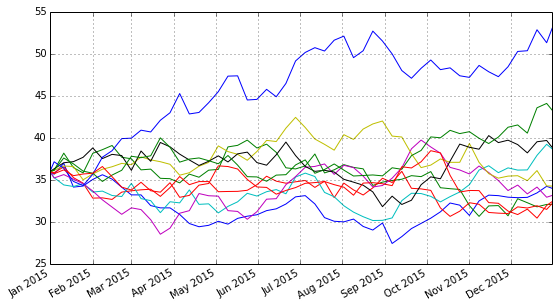Second, the American put option.

In :
me_put = dx.market_environment('put', gbm.pricing_date)
put = dx.valuation_mcs_american_single(
'put', gbm, me_put,
payoff_func='np.maximum(strike - instrument_values, 0)')


Valuation and Greeks for the American put.

In :
put.present_value()

Out:
4.432783

In :
put.delta()

Out:
-0.6757

In :
put.vega()

Out:
11.5551


Some sensitivities of the American put (I).

In :
%%time
s_list = np.arange(32., 50.1, 2.)
pv = []; de = []; ve = []
for s in s_list:
put.update(initial_value=s)
pv.append(put.present_value())
de.append(put.delta())
ve.append(put.vega())
put.update(initial_value=36.)

CPU times: user 31 s, sys: 3.98 s, total: 34.9 s
Wall time: 34.7 s



Some sensitivities of the American put (II).

In :
dx.plot_option_stats(s_list, pv, de, ve)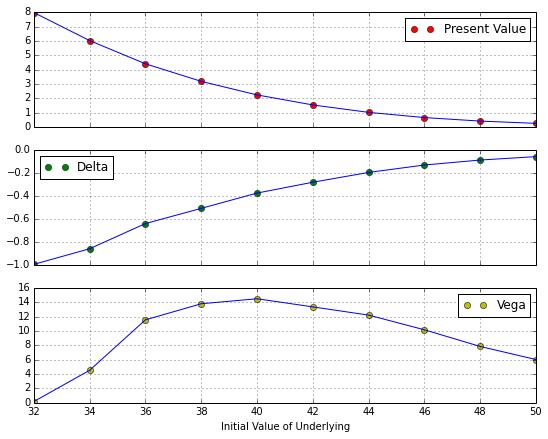### European Maximum Call Option¶

Now a more complex, two risk factor European option.

In :
%run dx_example.py
# sets up market environments
# and defines derivative instrument

In :
max_call.payoff_func
# payoff of a maximum call option
# on two underlyings (European exercise)

Out:
"np.maximum(np.maximum(maturity_value['gbm'], maturity_value['jd']) - 34., 0)"


We are going to generate a Vega surface for one risk factor with respect to the initial values of both risk factors.

In :
asset_1 = np.arange(28., 46.1, 2.)
asset_2 = asset_1
a_1, a_2 = np.meshgrid(asset_1, asset_2)
value = np.zeros_like(a_1)

In :
%%time
vega_gbm = np.zeros_like(a_1)
for i in range(np.shape(vega_gbm)):
for j in range(np.shape(vega_gbm)):
max_call.update('gbm', initial_value=a_1[i, j])
max_call.update('jd', initial_value=a_2[i, j])
vega_gbm[i, j] = max_call.vega('gbm')

CPU times: user 4.47 s, sys: 250 ms, total: 4.72 s
Wall time: 4.72 s



The results visualized.

In :
dx.plot_greeks_3d([a_1, a_2, vega_gbm], ['gbm', 'jd', 'vega gbm'])
# Vega surface plot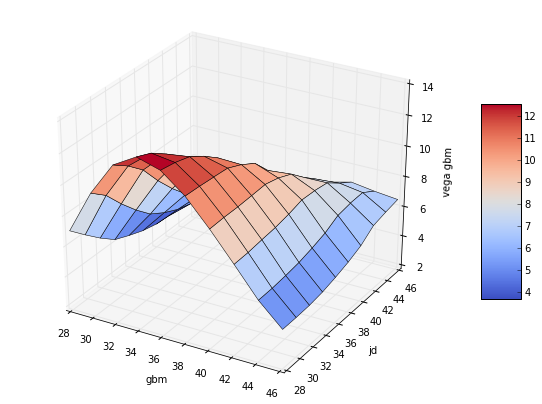In June 2013, Eurex launched the VSTOXX Advanced Services, a series of Python-based tutorials about the VSTOXX and related derivatives. Contents covered:

• Python Preliminaries
• Analyzing Historical VSTOXX Data
• Calculating the VSTOXX Index
• Valuing Volatility Derivatives
• Automated Monte Carlo Tests
• Calibration of the Pricing Model
• Backtesting of VSTOXX Strategies

## Python Quant Platform¶

The Python Quant Platform is developed and maintained by The Python Quants. It offers Web-/browser-based data and financial analytics for individuals, teams and organizations. Free registrations are possible under http://trial.quant-platform.com.

At the moment, the Python Quant Platform comprises the following components and features:

• IPython Notebook: interactive data and financial analytics in the browser with full Python integration and much more (cf. IPython home page).
• Anaconda Python Distribution: complete Python stack for financial, scientific and data analytics workflows/applications (cf. Anaconda page); you can easily switch between Python 2.7 and 3.4.
• DX Analytics: our library for advanced financial and derivatives analytics with Python based on Monte Carlo simulation.
• File Manager: a GUI-based File Manager to upload, download, copy, remove, rename files on the platform.
• Chat/Forum: there is a simple chat/forum application available via which you can share thoughts, documents and more.
• Collaboration: the platform features user/group administration as well as file sharing via public folders.
• Linux Server: the platform is powered by Linux servers to which you have full shell access (eg for IPython shell, Vim editing, Git integration).
• Deployment: the platform is easily scalable since it is cloud-based and can also be easily deployed on your own servers (via Docker containers).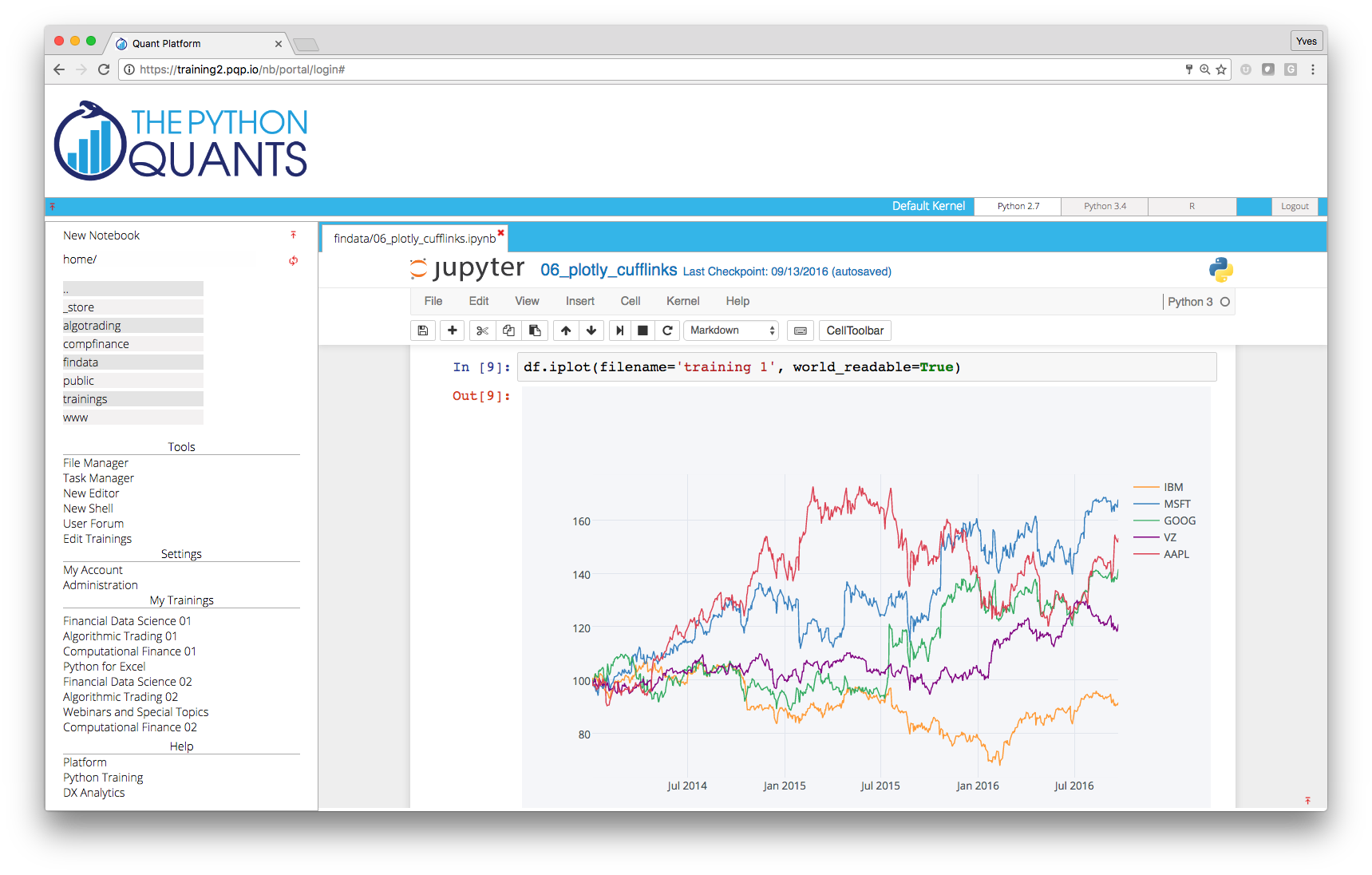IPython Notebook provides a unified analytics environment for a couple of (domain specific) languages, like R.

In :
%load_ext rpy2.ipython

In :
X = np.arange(100)
Y = 2 * X + 5 + 2 * np.random.standard_normal(len(X))
%Rpush X Y


Plotting with R from within IPython.

In :
%R plot(X, Y, pch=19, col='blue'); grid(); title("R Plot with IPython")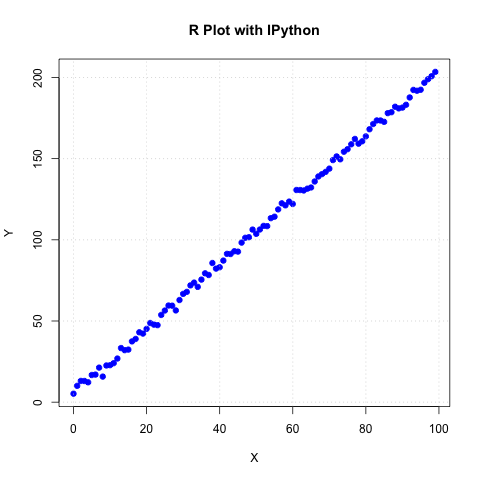Doing statistics with R.

In :
%R print(summary(lm(Y~X)))


Call:
lm(formula = Y ~ X)

Residuals:
Min      1Q  Median      3Q     Max
-5.6227 -1.1901  0.0544  1.4933  4.5572

Coefficients:
Estimate Std. Error t value Pr(>|t|)
(Intercept) 5.429466   0.399013   13.61   <2e-16 ***
X           1.992930   0.006963  286.20   <2e-16 ***
---
Signif. codes:  0 ‘***’ 0.001 ‘**’ 0.01 ‘*’ 0.05 ‘.’ 0.1 ‘ ’ 1

Residual standard error: 2.01 on 98 degrees of freedom
Multiple R-squared:  0.9988,	Adjusted R-squared:  0.9988
F-statistic: 8.191e+04 on 1 and 98 DF,  p-value: < 2.2e-16



Pulling data from R to Python.

In :
%R c = coef(lm(Y~X))
%Rpull c
c

Out:
<FloatVector - Python:0x10efdb170 / R:0x1016ee6a8>
[5.429466, 1.992930]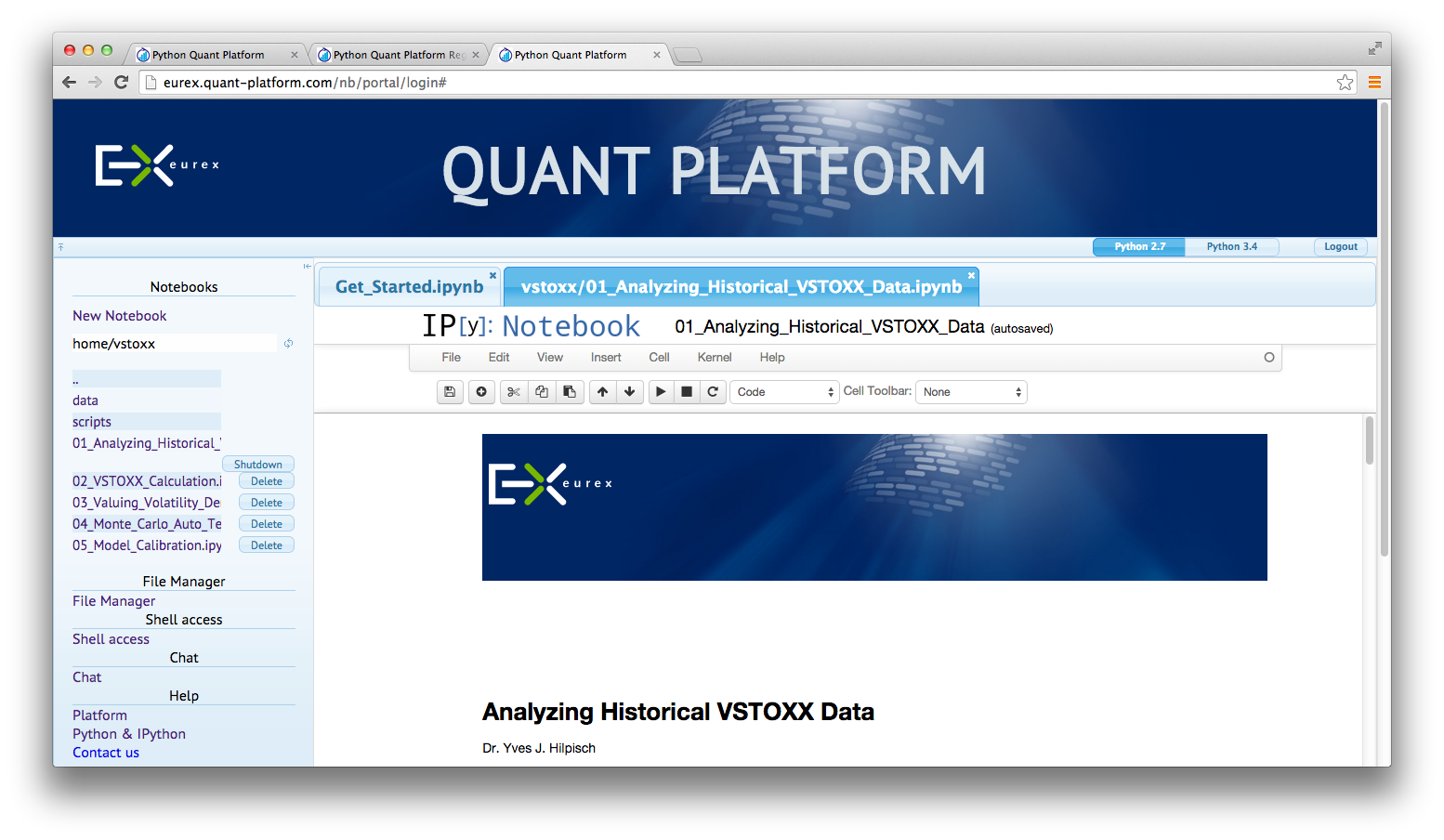## VSTOXX Options Pricing¶

### Vol-Vol Surface¶

VSTOXX implied vol-vol on 28. Dezember 2012.VSTOXX implied vol-vol on 31. March 2014.### Square-Root Diffusion¶

One of the early models for volatility option pricing has been the square-root diffusion process (SRD) as proposed by Gruenbichler Longstaff (1996):

$$dv_{t}=\kappa (\theta -v_{t})dt+\sigma \sqrt{v_{t}}dZ_{t}$$

The meaning of the variables and parameters is

• $v_{t}$ volatility index level at date $t$
• $\kappa$ speed of adjustment of $v_{t}$ to ...
• $\theta$, the long-term mean of the index
• $\sigma$ volatility coefficient of the index level
• $Z_{t}$ standard Brownian motion

Using DX Analytics, we calibrate the SRD model to European call options on the VSTOXX. The time series data is from QI of 2014. We calibrate to 2 maturities:

• April 2014 (shortest one with data available throughout)
• August 2014 (longest one with data available throughout)### Square-Root Jump Diffusion¶

To better account for the vol-vol smile implied in volatility option prices as well as for jumps, we add a log-normal jump compnent to the SRD process to end up with a square-root jump diffusion (SRJD):

$$dv_{t}=\kappa (\theta -v_{t})dt+\sigma \sqrt{v_{t}}dZ_{t} + J_{t} v_{t} dN_{t} -r_{J}dt$$

• $J_{t}$ jump at date $t$ with distribution ...
• ... $\log(1+J_{t})\approx \mathbf{N}\left(\log(1+\mu)-\frac{\delta^{2}}{2},\delta^{2}\right)$
• $\mathbf{N}$ cumulative distribution function of a standard normal random variable
• $N_{t}$ Poisson process with intensity $\lambda$
• $r_{J}\equiv \lambda \cdot \left(e^{\mu + \delta^{2}/2}-1\right)$ drift correction for jump### Square Root Jump Diffusion Plus¶

Taking the term structure, i.e. futures prices, into account and calibrating the model to multiple maturities simulataneously. Cf. CIR+ model by Brigo Mercurio (2001).Calibration over all maturities with +/-40% otm/itm options (total of 129 options).To be launched rather soon with the following topics covered:

• Realized Variance and Variance Swaps
• Model-Free Replication of Variance
• Variance Futures at Eurex